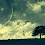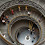> Fresco Play Python Pandas Hands- on Solution || T Factor - TECH UPDATE

# Fresco Play Python Pandas Hands-on Solution - T Factor (Course ID:- 55937)

In Python Pandas(Course Id:- 55937), There are 8 Hands-On Questions Available. The Solutions are

####### Code:-

import pandas as pd
import numpy as np
heights_A = pd.Series([176.2,158.4,167.6,156.2,161.4])
heights_A.index = ['s1','s2','s3','s4','s5']
print(heights_A.shape)

weights_A = pd.Series([85.1,90.2,76.8,80.4,78.9])
weights_A.index = ['s1','s2','s3','s4','s5']
print(weights_A.dtype)

df_A = pd.DataFrame()
df_A['Student_height'] = heights_A
df_A['Student_weight'] = weights_A
print(df_A.shape)

my_mean = 170.0
my_std = 25.0
np.random.seed(100)
heights_B = pd.Series(np.random.normal(loc = my_mean, scale = my_std, size = 5))
heights_B.index = ['s1','s2','s3','s4','s5']

my_mean1 = 75.0
my_std1 = 12.0
weights_B = pd.Series(np.random.normal(loc = my_mean1,scale = my_std1,size = 5))
weights_B.index = ['s1','s2','s3','s4','s5']
print(heights_B.mean())

df_B = pd.DataFrame()
df_B['Student_height'] = heights_B
df_B['Student_weight'] = weights_B
print(df_B.columns)

data = {'ClassA' : df_A,'ClassB':df_B}
p = pd.Panel.from_dict(data)
print(p.shape)

### 2. Data Cleaning Solutions - Python Pandas

Code:-
import pandas as pd
import numpy as np
height_A = pd.Series([176.2,158.4,167.6,156.2,161.4])
height_A.index = ['s1','s2','s3','s4','s5']
weight_A = pd.Series([85.1,90.2,76.8,80.4,78.9])
weight_A.index = ['s1','s2','s3','s4','s5']
df_A = pd.DataFrame()
df_A['Student_height'] = height_A
df_A['Student_weight'] = weight_A

df_A.loc['s3'] = np.nan
df_A.loc['s5'] = np.nan

df_A2 = df_A.dropna(how = 'any')
print(df_A2)

### 3. Data Merging Hands-On(2) Solution: Python Pandas

#### Code:-

import pandas as pd
import numpy as np

height_A = pd.Series([176.2,158.4,167.6,156.2,161.4])
height_A.index = ['s1','s2','s3','s4','s5']

weights_A = pd.Series([85.1,90.2,76.8,80.4,78.9])
weights_A.index = ['s1','s2','s3','s4','s5']

df_A = pd.DataFrame()
df_A['Student_height'] = height_A
df_A['Student_weight'] = weights_A

df_A['Gender'] = ['M','F','M','M','F']

s = pd.Series([165.4,82.7,'F'],index = ['Student_height','Student_weight','Gender'],name='s6')

df_AA = df_A.append(s)
print(df_AA)

my_mean = 170.0
my_std = 25.0
np.random.seed(100)
heights_B = pd.Series(np.random.normal(loc = my_mean,scale=my_std,size = 5))
heights_B.index = ['s1','s2','s3','s4','s5']

my_mean1 = 75.0
my_std1 = 12.0
np.random.seed(100)
weights_B = pd.Series(np.random.normal(loc = my_mean1,scale=my_std1,size = 5))
weights_B.index = ['s1','s2','s3','s4','s5']

df_B = pd.DataFrame()
df_B['Student_height'] = heights_B
df_B['Student_weight'] = weights_B

df_B.index=['s7','s8','s9','s10','s11']
df_B['Gender'] = ['F','M','F','F','M']

df = pd.concat([df_AA,df_B])
print(df)

### 4. Data Merging Hands-On(1) Solutions:- Python Pandas

#### Code:-

import pandas as pd
import numpy as np
nameid = pd.Series(range(101,111))
name = pd.Series(['person' + str(i) for i in range(1,11)])
master = pd.DataFrame()
master['nameid'] = nameid
master['name'] = name
transaction = pd.DataFrame({'nameid':[108,108,108,103],'product':['iPhone','Nokia','Micromax','Vivo']})
mdf = pd.merge(master,transaction,on='nameid')
print(mdf)

### 5. Indexing Dataframe Hands-On Solutions - Python Pandas

Code:-
import pandas as pd
import numpy as np

DatetimeIndex = pd.date_range(start = '09/01/2017',end='09/15/2017')
print(DatetimeIndex)

datelist = ['14-Sep-2017','09-Sep-2017']
date_to_be_searched = pd.to_datetime(datelist)
print(date_to_be_searched)

print(date_to_be_searched.isin(datelist))

arraylist = [['classA']*5 + ['classB']*5,['s1','s2','s3','s4','s5']* 2]
mi_index = pd.MultiIndex.from_product(arraylist,names=['First Level','Second Level'])
print(mi_index.levels)

### 6.Data Aggression:- Python Pandas

#### Code:-

import pandas as pd
import numpy as np
heights_A = pd.Series([176.2,158.4,167.6,156.2,161.4])
heights_A.index = ['s1','s2','s3','s4','s5']
weights_A = pd.Series([85.1,90.2,76.8,80.4,78.9])
weights_A.index = ['s1','s2','s3','s4','s5']

df_A = pd.DataFrame()

df_A['Student_height'] = heights_A
df_A['Student_weight'] = weights_A

df_A_filter1 = df_A[(df_A.Student_weight < 80.0) & (df_A.Student_height > 160.0)]
print(df_A_filter1)

df_A_filter2 = df_A[df_A.index.isin(['s5'])]
print(df_A_filter2)

df_A['Gender'] = ['M','F','M','M','F']
df_groups = df_A.groupby('Gender')
print(df_groups.mean())

### 7. Accessing Pandas Data Structures - Python Pandas

Code:-

import pandas as pd
import numpy as np

heights_A = pd.Series([176.2,158.4,167.6,156.2,161.4])
heights_A.index = ['s1','s2','s3','s4','s5']
print(heights_A)

print(heights_A[1:4])

weights_A = pd.Series([85.1,90.2,76.8,80.4,78.9])
weights_A.index = ['s1','s2','s3','s4','s5']

df_A = pd.DataFrame()
df_A['Student_height'] = heights_A
df_A['Student_weight'] = weights_A

height = df_A['Student_height']
print(type(height))

df_s1s2 = df_A[df_A.index.isin(['s1','s2'])]
print(df_s1s2)

df_s2s5s1 = df_A[df_A.index.isin(['s1','s2','s5'])]
df_s2s5s1 = df_s2s5s1.reindex(['s2','s5','s1'])
print(df_s2s5s1)

df_s1s4 = df_A[df_A.index.isin(['s1','s4'])]
print(df_s1s4)

### Code:-

import pandas as pd
import numpy as np
heights_A = pd.Series([176.2,158.4,167.6,156.2,161.4])
heights_A.index = ['s1','s2','s3','s4','s5']
weights_A = pd.Series([85.1,90.2,76.8,80.4,78.9])
weights_A.index = ['s1','s2','s3','s4','s5']
df_A = pd.DataFrame()
df_A['Student_height'] = heights_A
df_A['Student_weight'] = weights_A
df_A.to_csv('classA.csv')

print(df_A2)

print(df_A3)

my_mean = 170.0
my_std = 25.0
np.random.seed(100)
heights_B = pd.Series(np.random.normal(loc = my_mean, scale = my_std, size = 5))
heights_B.index = ['s1','s2','s3','s4','s5']
my_mean1 = 75.0
my_std1 = 12.0
np.random.seed(100)
weights_B = pd.Series(np.random.normal(loc = my_mean1,scale = my_std1,size = 5))
weights_B.index = ['s1','s2','s3','s4','s5']
df_B = pd.DataFrame()
df_B['Student_height'] = heights_B
df_B['Student_weight'] = weights_B

df_B.to_csv('classB.csv',index = False)
print('classB.csv')

print(df_B2)

print(df_B3)

print(df_B4)

## Thank you

Fresco Play Python Pandas Hands- on Solution || T FactorReviewed by TECH UPDATE on March 08, 2021 Rating: 5

1.1.Bro data merging 1 executed for u now

2.mdf = mdf[['nameid','name','product']]

2.Numpy hands-on answers kooda pettu bro :)

3.can anyone put hands on numpy also for fresco play!! plz

4.machine learning handson also upload bro

5.Hi , I am unable to clear Accessing Pandas Data Structures - Python Pandas, it's just saying not passed validation in tasks,5,6 it has asked to use .loc or.iloc as well. I have used that too.can you help

6.Data Merging Handson-2 is getting executed correctly in Hackerank but in fresco results are not updated.

1.Can you please tell me how to solve , I'm also facing same issue

2.Even i am facing similar issue. can someone help how to solve this issue

3.even i got that issue. can someone help me in this issue

4.Anyone fixed this am also getting the same

7.Can you please provide hands on for node.js essential fron fresco

8.This comment has been removed by a blog administrator.

9.not useful

10.Data merging hands-on-1 is not passing preliminary validations can u help out??

1.import pandas as pd

import numpy as np

height_A = pd.Series([176.2,158.4,167.6,156.2,161.4])

height_A.index = ['s1','s2','s3','s4','s5']

weights_A = pd.Series([85.1,90.2,76.8,80.4,78.9])

weights_A.index = ['s1','s2','s3','s4','s5']

df_A = pd.DataFrame()

df_A['Student_height'] = height_A

df_A['Student_weight'] = weights_A

df_A['Gender'] = ['M','F','M','M','F']

s = pd.Series([165.4,82.7,'F'],index = ['Student_height','Student_weight','Gender'],name='s6')

df_AA = df_A.append(s)

print(df_AA)

my_mean = 170.0

my_std = 25.0

np.random.seed(100)

heights_B = pd.Series(np.random.normal(loc = my_mean,scale=my_std,size = 5))

heights_B.index = ['s1','s2','s3','s4','s5']

my_mean1 = 75.0

my_std1 = 12.0

np.random.seed(100)

weights_B = pd.Series(np.random.normal(loc = my_mean1,scale=my_std1,size = 5))

weights_B.index = ['s1','s2','s3','s4','s5']

df_B = pd.DataFrame()

df_B['Student_height'] = heights_B

df_B['Student_weight'] = weights_B

df_B.index=['s7','s8','s9','s10','s11']

df_B['Gender'] = ['F','M','F','F','M']

df = pd.concat([df_AA,df_B])

print(df)

2.Thank u so much

3.The NP and PD files import are showing import error how to import those in the hacker rank

11.Thank you ! Finally a vid that shows what you are doing and doesnt click on the icons and buttons rlly fast so you cant see what they're doin, as if they're trying to impress the viewers with how fast they can type och click... annoying. But I loved this(:﻿
Jayme Silvestri

12.7.Welcome to Python Pandas | 8 | Data Merging 2(75 Min)

Data Merge - Hands-on 2

File Name: prog.py

import pandas as pd

import numpy as np

nameid = pd.Series(range(101,111))

name = pd.Series(['person' + str(i) for i in range(1,11)])

master = pd.DataFrame()

master['nameid'] = nameid

master['name'] = name

transaction = pd.DataFrame({'nameid':[108,108,108,103],'product':['iPhone','Nokia','Micromax','Vivo']})

mdf = pd.merge(master,transaction,on='nameid')

print(mdf)

1.13.6.Welcome to Python Pandas | 7 | Data Merging 1(75 Min)

Data Merge - Hands-on 1

File Name: prog.py

import pandas as pd

import numpy as np

height_A = pd.Series([176.2,158.4,167.6,156.2,161.4])

height_A.index = ['s1','s2','s3','s4','s5']

weights_A = pd.Series([85.1,90.2,76.8,80.4,78.9])

weights_A.index = ['s1','s2','s3','s4','s5']

df_A = pd.DataFrame()

df_A['Student_height'] = height_A

df_A['Student_weight'] = weights_A

df_A['Gender'] = ['M','F','M','M','F']

s = pd.Series([165.4,82.7,'F'],index = ['Student_height','Student_weight','Gender'],name='s6')

df_AA = df_A.append(s)

print(df_AA)

my_mean = 170.0

my_std = 25.0

np.random.seed(100)

heights_B = pd.Series(np.random.normal(loc = my_mean,scale=my_std,size = 5))

heights_B.index = ['s1','s2','s3','s4','s5']

my_mean1 = 75.0

my_std1 = 12.0

np.random.seed(100)

weights_B = pd.Series(np.random.normal(loc = my_mean1,scale=my_std1,size = 5))

weights_B.index = ['s1','s2','s3','s4','s5']

df_B = pd.DataFrame()

df_B['Student_height'] = heights_B

df_B['Student_weight'] = weights_B

df_B.index=['s7','s8','s9','s10','s11']

df_B['Gender'] = ['F','M','F','F','M']

df = pd.concat([df_AA,df_B])

print(df)

14.15.Accessing pandas Data Structures
import errors are coming. what can I do now?

16.import pandas as pd
import numpy as np

Here import is error occurred how to resolve it
I try it my level best but i didn't clear

1.we can't use the pandas in that program

17.Working with c

18.19.